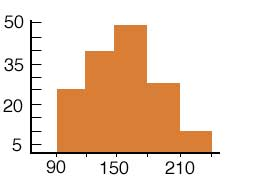SEARCH HOMEMath Central Quandaries & QueriesBea, a student: From a histogram only, can you find the frequency per each increment of an interval?Hi Bea,

A histogram is actually a graph of tabulated frequencies. Along the horizontal axis is the intervals and along the vertical axis is the frequencies.For example: In the above histogram, the interval of 90-120 has a frequency of 25.

Hope this helps,

JaniceMath Central is supported by the University of Regina and The Pacific Institute for the Mathematical Sciences.